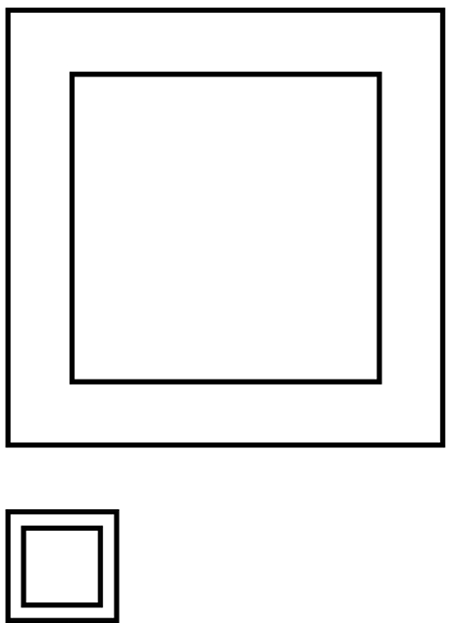## on amountsThe area of the above squares shall visualize a certain amount of money.

A little puzzle for randform visitors:

The upper outer square visualizes an amount which is … times bigger as the amount of the upper inner square.

The lower outer square visualizes an amount which is … times bigger as the amount of the lower inner square.

solution:

If inkscape doesnt have a bug then the amount which is visualized by the area of the outer square should be in both cases twice as big as the amount visualized by the inner square. I.e. if the inner square visualizes 300 billion \$ then the outer square visualizes 600 billion \$.

more precise: the upper inner square has an area of 4*4 units and thus the upper outer square has an area of 4*4*2 units, the lower inner square an area of 1*1 units.

The below box is for leaving comments. Interesting comments in german, french and russian will eventually be translated into english. If you write a comment you consent to our data protection practices as specified here. If your comment text is not too rude and if your URL is not clearly SPAM then both will be published after moderation. Your email adress will not be published. Moderation is done by hand and might take up to a couple of days.
you can use LaTeX in your math comments, by using the [latex] shortcode:
[latex] E = m c^2 [/latex]